# Catch up on the latest AI articles# Should Feature Expressions Be Low-dimensional?

3 main points
✔️ General representation learning acquires low-dimensional representations from high-dimensional inputs.
✔️ A proposal for a method of obtaining a high-dimensional representation from a low-dimensional input that defies stereotypes
✔️ Improved performance of continuous control tasks when combined with existing reinforcement learning

Can Increasing Input Dimensionality Improve Deep Reinforcement Learning?
writte
(Submitted on 3 Mar 2020 (v1), last revised 27 Jun 2020 (this version, v2))

Subjects: Machine Learning (cs.LG); Robotics (cs.RO); Machine Learning (stat.ML)
Paper
Official Code COMM Code

## Introduction

The most critical problem in deep learning is representation learning, which extracts important features from the raw input data and obtains latent representations that are useful for solving the task.

For example, let's say you want the AI to play a block-busting game. In this case, the input data would be RGB images of tens to hundreds of pixels in height and width, and the number of input dimensions would be in the thousands to tens of thousands. Deep reinforcement learning does not use such high-dimensional data as it is. First, important features are extracted using CNN and so on, and then transformed into a latent representation with fewer dimensions. This low-dimensional representation is then used for effective learning. Intuitively, the key to solving block collapses would be the position of the block, the ball, and the bar to be manipulated.

In other words, high-dimensional input has many problems in using it to solve tasks, such as containing a lot of unnecessary data and not organizing important information. That is why it is important to use representational learning that can properly obtain the information needed to solve tasks. Typical representation learning retrieves low-dimensional latent representations from high-dimensional input data. As in the previous example, it is mostly about transforming unwieldy high-dimensional data into low-dimensional, tractable data.

So what happens if, on the other hand, you get a high-dimensional latent representation from low-dimensional input data?

In other words, are high-dimensional latent representations for inputs useful in solving tasks?

The paper presented here is a study that provides an affirmative answer to this question about representational learning in deep reinforcement learning.

## OFENet (Proposal Methodology)

In reinforcement learning, the agent receives observations $o_t$ and rewards $r_t$ from the environment at some time $t$ and chooses an action $a_t$.

The method proposed in the paper, Online Feature Extractor Network (OFENet), takes observations $o_t$ and actions $a_t$ as inputs and obtains a high-dimensional observation representation $z_{o_t}$ and an observation-action pair representation $z_{o_t,a_t}$.

When we actually perform the reinforcement learning task, we use a separate reinforcement learning algorithm from OFENet.

However, this reinforcement learning algorithm uses $z_{o_t}$,$z_{o_t,a_t}$ obtained by OFENet instead of the observations $o_t$ and actions $a_t$.

## Architecture

The architecture of OFENet is as follows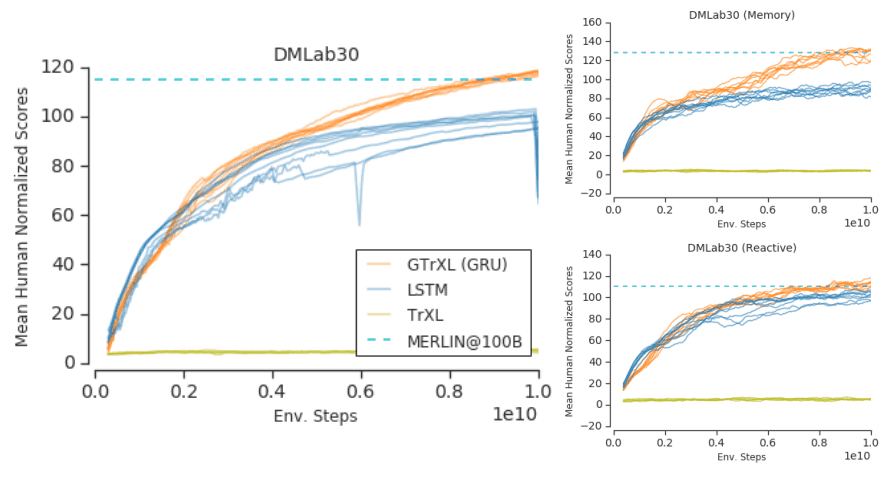As shown in the figure, the observation $o_t$ is transformed into the observation representation $z_{o_t}$ by the map $φ_o$ with the parameter $θ_{φ_o}$, and the action $a_t$ is transformed into the observation representation $z_{o_t}$ by the map $φ_o$ with the parameter $θ_{φ_{o,a}}$. Also, the observation representation $z_{o_t}$ and the action $a_t$ are transformed into the observation-action pair representation $z_{o_t,a_t}$ by the map $φ_{o,a}$ with the parameter $θ_{φ_{o,a}}$.

This can be expressed as a formula as follows

$z_{o_t} = φ_o(o_t)$

$z_{o_t,a_t} = φ_{o,a}(o_t,a_t)$

These mappings $φ_o$,$φ_{o,a}$ utilize a DenseNet-tuned MLP-DenseNet.

In MLP-DenseNet, the output $y$ of each layer is $y = [x, σ(W_1x+b)]$, which is the concatenation of the input $x$ with the product of the weight matrix $W_1$ and the input $x$ ($[x1,x2]$ is the concatenation and $σ$ is the activation function. Bias is omitted).

In other words, the convolutional operations of DenseNet are replaced by a MultiLayer Perceptron. So, as with DenseNet, the inputs and outputs of the lower layers are included in the final output. In our experiments, in addition to MLP-DenseNet and MLP (the usual multilayer perceptron), we also used a ResNet-tuned MLP-ResNet, which, like MLP-DenseNet, replaces the ResNet convolutional operations with a multilayer perceptron format.

## Distribution Shift

FENet is trained simultaneously with the reinforcement learning algorithm. Therefore, as OFENet is trained, the distribution of input to the reinforcement learning algorithm may change. This is called distribution shift and is known to be a serious problem in reinforcement learning. (AI-SCHOLAR mentions it in this and other articles ).

To mitigate this problem, we use Batch Normalization to suppress the change in distribution.

The goal of a reinforcement learning algorithm is to learn measures that maximize the cumulative reward. OFENet needs to learn high-dimensional state and behavioral representations to help it learn such measures. To do so, we use an auxiliary task that takes the observation/behavioral representation $z_{o_t}$,$a_t$ as input and predicts the next observation $o_{t+1}$.

This auxiliary task is performed by the module $f_{pred}$ with the parameter $θ_{pred}$, where $f_{pred}$ is represented as a linear combination of $z_{o_t}$ and $a_t$. where $f_{pred}$ is represented as a linear combination of observation and action representations $z_{o_t}$,$a_t$.

We train the reinforcement learning algorithm and OFENet at the same time. That is, we optimize the OFENet parameter $θ_{aux} = \{θ_{pred}, θ_{φ_o} , θ_{φ_{o,a}} \}$ so that the auxiliary task loss $L_{aux}$ shown below is minimized.

$L_{aux} = E_{(ot,at)∼p,π}[||f_{pred}(z_{o_t},a_t ) - o_{t+1}||^2 ]$

In order to get the full performance of OFENet, it is necessary to choose the right hyperparameters. However, optimal hyperparameters are strongly dependent on the environment and the reinforcement learning algorithm.

Hence, we need an efficient way to find the effective hyperparameters. Since it is inefficient to perform a reinforcement learning task and compare the performance, we use the performance of the auxiliary task to determine the hyperparameters.

The procedure for determining the performance of the auxiliary task is as follows

• We collect the history of observations and actions by executing random measures and randomly divide them into train and test sets.
• After training in the train set, we use the five random seeds in the test set to find the average auxiliary loss $L_{aux}$.
• The architecture with the lowest average auxiliary loss is used in the actual task.

In this way, the hyperparameters are efficiently determined without actually having the reinforcement learning agent interact with the environment to learn.

## Experimental results

In our experiments, we verify the performance based on a continuous control task where the input is low-dimensional.

All experiments are conducted in the MuJoCo environment.

### comparative study

First, the results of the comparison between the case with and without the representation obtained by OFENet (original) are shown below.

As mentioned earlier, OFENet is used in combination with existing reinforcement learning algorithms. The algorithms used here for comparison are as follows

In all cases, $z_{o_t}$,$z_{o_t,a_t}$ is set to 240 dimensions larger than the input dimension.

Also, instead of OFENet, we use a representation generated using ML-DDPG for comparison.

In this case, we combine ML-DDPG and SAC (corresponding to ML-SAC in the following figure). The number of dimensions of the representation is examined both for the original case (1/3 of the input dimension) and for the same dimension as OFENet (OFE like).

The learning curve and the highest average returns for the five random seeds are as follows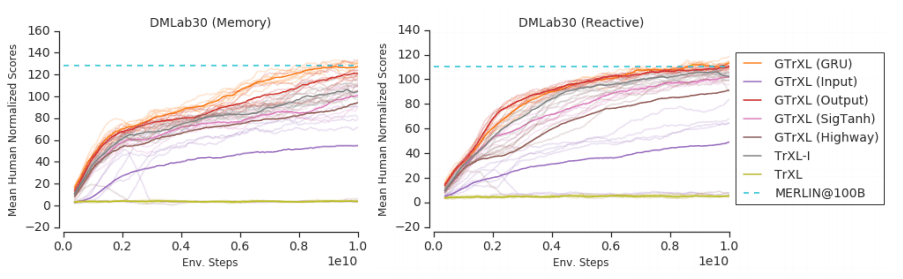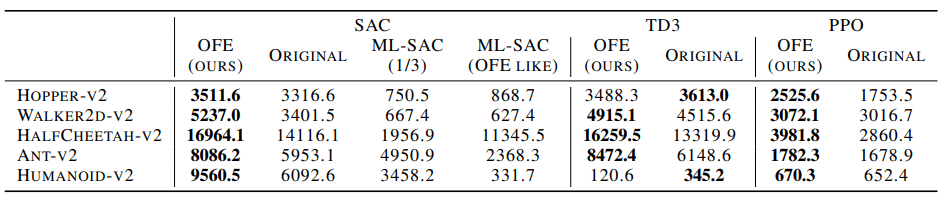As it can be seen from the figures and tables, the performance of ML-SAC was higher than that of ML-DDPG, an existing method of representation learning (ML-SAC), and that of the original method without MFE (original).

## Ablation Studies

OFENet used MLP-DenseNet, which replaces the DenseNet convolutional layer with a multilayer perceptron. Instead of this MLP-DenseNet, MLP-ResNet and MLP would look like the following figure.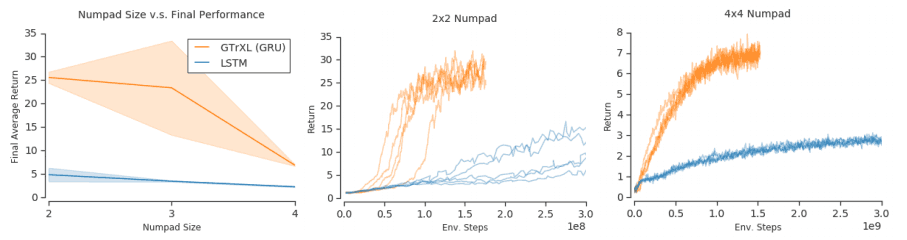As you can see in this figure, the most outstanding performance is seen when using MLP-DenseNet. It is not easy to properly construct a high-dimensional representation, as network changes have a very significant impact on performance.

In order to examine other elements of OFENet's architecture that contribute to performance improvement, we conduct ablation studies on the following examples. We use SAC for the reinforcement learning algorithm and Ant-v2 for the simulation environment.

full: when the full OFENet representation is used.
original: Original SAC
no-bn: No Batch Normalization
The same number of SAC parameters as OFENet.
Freeze-ofe: Train and fix the OFENet first, then train the reinforcement learning algorithm.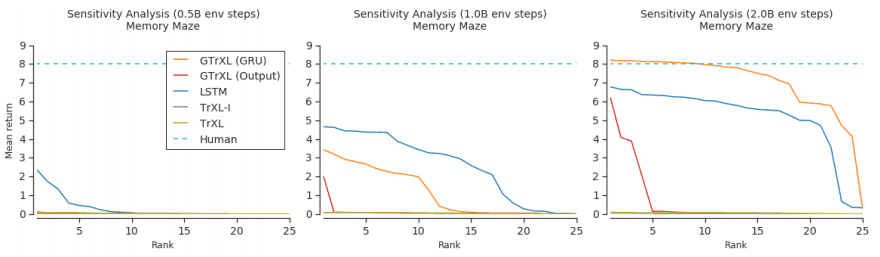As shown in the figure, the simultaneous training of the auxiliary task, Batch Normalization, and the OFENet and reinforcement learning algorithms contribute to the performance improvement of OFENet.

We also examine how increasing the number of dimensions of the OFENet representation affects the performance of reinforcement learning. The following figure shows the case of varying the number of dimensions of $z_o$,$z_{o,a}$.

Thus, we found that the performance improved up to a certain threshold as the number of dimensions increased.

## Summary

In general representation learning, a low-dimensional latent representation is obtained from a high-dimensional input. However, as we have shown here, it was shown that reinforcement learning agents may be able to perform better by converting low-dimensional inputs to high-dimensional representations.

This is a very important and promising study that throws a stone at the stereotypes of expression learning.

## Recommendations for similar papers

Deep Reinforcement Learning for Robotic Manipulation with Asynchronous Off-Policy Updates
written by Shixiang GuEthan HollyTimothy LillicrapSergey Levine
(Submitted on 3 Oct 2016 (v1), last revised 23 Nov 2016 (this version, v2))

Subjects: Robotics (cs.RO); Artificial Intelligence (cs.AI); Machine Learning (cs.LG)
Paper
Official Code COMM Code

Agent Modeling as Auxiliary Task for Deep Reinforcement Learning
written by Pablo Hernandez-LealBilal KartalMatthew E. Taylor
(Submitted on 22 Jul 2019)

Subjects:  Multiagent Systems (cs.MA); Machine Learning (cs.LG)
Paper
Official Code COMM Code

If you have any suggestions for improvement of the content of the article,